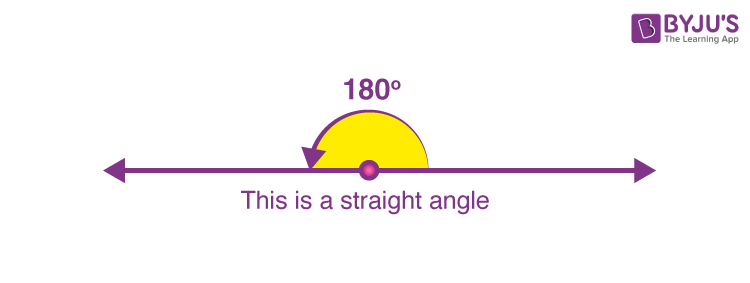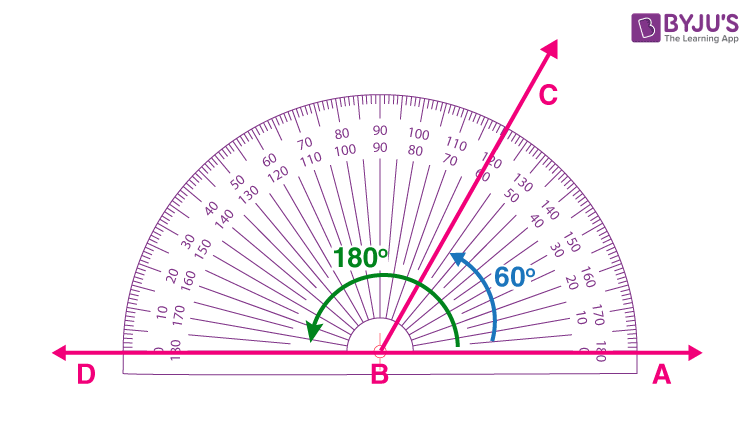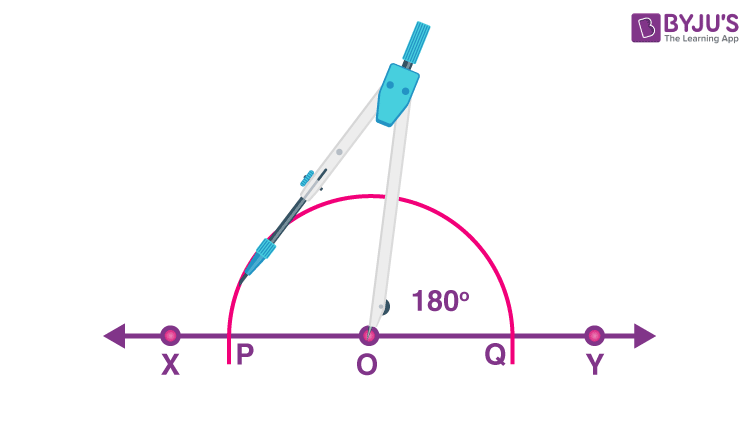# 180 Degree Angle

What is 180 degree angle?

The angle which forms a straight line is called the 180-degree angle. In geometry, we will be introduced to different types of angles, such as acute angle, obtuse angle, right angle, straight angle, reflex angle and full rotation. The angle which measures 180 degrees is named the straight angle. The other angles are given here:

• Acute angle: The angle which is more than 0° and less than 90°
• Right angle: The angle which is equal to 90°
• Obtuse angle: The angle which is more than 90° but less than 180°
• Reflex angle: The angle which is more than 180°
• Full rotation angle: The angle equal to 360°In this article, you will learn how to measure the angles 180 degrees using a protractor, how to construct the 180 degree angle using a compass along with steps and construction images.

### 180 Degree Angle Name

The arms which form 180 degree angle is a straight line which can be observed from the below figure and thus, 180 degree angle is known as straight angle.## How to Find 180-Degree Angle?

To measure any angle we can directly use a protractor or a compass. Here are the steps to find a 180° angle, given in a plain sheet.

• Draw a straight line which will be the arm of the angle.
• Put a dot at one end of the line. This dot denotes the vertex of the angle
• Now place the center of the protractor above the dot or vertex and match the baseline of the protractor along with the arm of the angle.
• Mark the angle 180 degrees with a dot.
• Now join this dot to the vertex of the angle, which forms the second arm of the angle.

Hence, we get a straight angle.### How to Draw a 180-Degree Angle Using a Compass?

To draw the straight angle using a compass follow the below steps.

• First, draw a straight line using a ruler or scale and name it as XY.
• Now mark a point O in anywhere between X and Y.
• With O as a center point, draw an arc of any radius using a compass, from the left of point O to the right of O.
• This arc cuts the straight line at point P and Q.
• Hence, the angle POQ is the required 180 degrees.Check: To check if the angle is 180 degree or not, you can use a protractor by keeping the center of the protractor at the top of point O and measuring the angle POQ.

### Table of Angles

As per the definition of different angles present in geometry, here is a brief description for each angle. Suppose θ is any angle, then;

 Type of angle Measure of angle Acute angle 0° < θ < 90° Right angle θ = 90° Obtuse angle 90° < θ < 180° Straight angle θ = 180° Reflex angle 180° < θ < 360° Full rotation (or Complete angle) θ = 360°

## Solved Examples

Q.1: If a straight angle is divided into two parts, and one angle measures 60 degrees, then find the other angle.

Solution: Let the unknown angle is x

Given, another angle = 60°

We know that;

Straight angle = 180°

Therefore,

x+60 = 180

x = 180 – 60

x = 120°

Hence, the other angle measures equal to 120°.

Q.2: Two angles are supplementary. If one angle is equal to 45 degrees, find the other angle.

Solution: Given, two angles are supplementary, thus the sum of the two angles forms a 180-degree angle.

Now given one angle is 45 degrees.

Let the other angle is x.

Thus,

45 + x = 180  {Supplementary angles adds up to 180 degrees}

x = 180 – 45 = 135 degrees

Q.3: If two angles are equal to each other and also supplementary, then find the angles.

Solution: Given, two angles are equal. Let the angles be x.

Also, given two angles are supplementary, therefore,

x + x = 180°

2x = 180°

x = 180/2

x = 90°

Therefore, both angles are 90° each.

Visit byjus.com to learn how to construct different angles and also download BYJU’S – The Learning App for more information on types of angles.

## Frequently Asked Questions – FAQs

Q1

### What is the 180-degree angle?

The 180-degree angle is called a straight angle.
Q2

### What does a 180-degree angle appear like?

A 180-degree angle looks like a straight line.
Q3

### How to find a 180-degree angle?

We can easily draw a 180-degree angle using a protractor or a compass.
Q4

### How many 90 degree angles are in a 180-degree angle?

Two 90 degrees angles add up to a straight angle.
Q5

### Is the straight angle and 180 -degree angle the same?

Yes, straight angle and 180-degree angle are the same.
Q6

### What are 180 degrees for a triangle?

For a triangle, the sum of all the interior angles is equal to 180 degrees. Also, the sum of each interior angle and exterior angle of a triangle is 180 degrees. Thus, the pair of an interior angle and exterior angle of a triangle results in a straight angle.
Q7

### What is the name of a 360-degree angle?

A 360-degree angle is called full angle or full rotation.